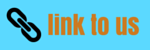# Light Class 8 Science Short Answer question CBSE

In this page we have Light Class 8 Science Short Answer question CBSE . Hope you like them and do not forget to like , social shar and comment at the end of the page.

## SHORT Answer Question

Question 1
What is virtual image?

Question 2
What are the characteristics of the image formed by a plane mirror?

Question 3
What is the function of a periscope in a submarine?

Question 4
Calculate the number of images formed by plane mirror kept an angle of 30o to each other?

Question 5
What is the main structural difference between a convex and a concave mirror?

Question 6
What is the aperture of a lens?

Question 7

For a proper in take of Vitamin A, what kind of food should you eat?

Question 8

What is the cause of refraction?

Question 9

Stars appear to be slightly higher in the sky than they actually are, Why is this so?

Question 10
Distinguish between near point and far point of eye?

Question 11
What are the differences between reflection and refraction of light?

Question 12
Give reasons-
a) A concave lens is also known as a diverging lens?
b) An object seen through a prison appears coloured.
c) It is not possible to see an object clearly if it is kept too close to the eye?
Question 13

Distinguish between converging and diverging lens?

Question 14
Why a pencil dipped in water appear bent at the surface of water?